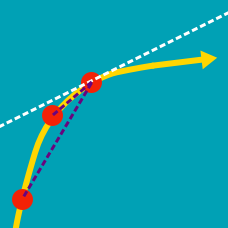Calculus

Discrete First Derivatives Warmup

$\begin{array} {c|c|c|c|c|c|c|c} n & 1 & 2 & 3 & 4 & 5 & 6 & \cdots \\ a_n = n^2 & \color{#D61F06}{1} & \color{#D61F06}{4} & \color{#D61F06}{9} & 16 & 25 & 36 & \cdots \\ \Delta(a_n) = a_{n+1} - a_n & \color{#3D99F6}{3} & \color{#3D99F6}{5} & ???\\ \end{array}$

The second row of the table above shows the first few terms of the sequence $a_n = n^2.$ Each entry in the third row is the difference between the next term in row 2 and the current term in row 2. For example, $\color{#3D99F6}{3} = \color{#D61F06}{4} -\color{#D61F06}{1}$ and $\color{#3D99F6}{5} = \color{#D61F06}{9} - \color{#D61F06}{4}.$ What number should go in the cell marked '???'?

$\begin{array} {c|c|c|c|c|c|c|c} n & 1 & 2 & 3 & 4 & 5 & 6 & \cdots \\ a_n = n^3 & 1 & 8 & 27 & 64 & 125 & 216 & \cdots \\ \Delta(a_n) = a_{n+1} - a_n & 7 & 19 & 37 & 61 & ???\\ \end{array}$

The second row of the table above shows the first few terms of the sequence $a_n = n^3.$ Each entry in the third row is the difference between the next term in row 2 and the current term in row 2. For example, $7 = 8 -1$ and $19 = 27 -8.$ What number should go in the cell marked '???'?

$\begin{array} {c|c|c|c|c|c|c|c} n & 1 & 2 & 3 & 4 & 5 & 6 & \cdots & n & n +1 \\ a_n = n^3 & 1 & 8 & 27 & 64 & 125 & 216 & \cdots &n^3 & (n+1)^3 \\ \Delta(a_n) = a_{n+1} - a_n & 7 & 19 & 37 & 61 & 91 & & \cdots & ? \\ \end{array}$

Given $a_n = n^3,$ find $\Delta(a_n)$.

In other words, what is the general formula for the discrete derivative of $\{n^3\}?$

Given $a_n = n^2 + n,$ find $\Delta(a_n),$ the discrete derivative of $\{a_n\}.$

Which of these sequences has a constant discrete derivative?

×